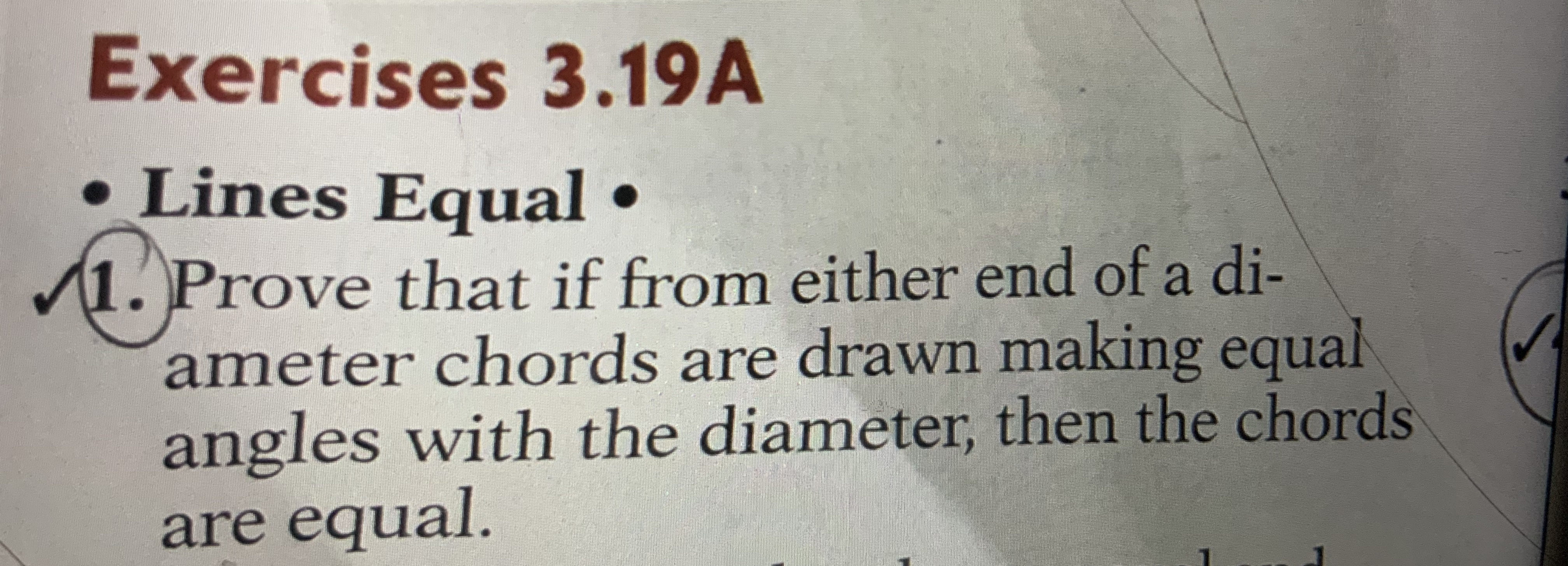# Exercises 3.19A• Lines Equal •1. Prove that if from either end of a di-ameter chords are drawn making equalangles with the diameter, then the chordsare equal.

Question
14 viewshelp_outlineImage TranscriptioncloseExercises 3.19A • Lines Equal • 1. Prove that if from either end of a di- ameter chords are drawn making equal angles with the diameter, then the chords are equal. fullscreen
check_circle

Step 1

Draw a circle. Let AB is the diameter.

AC and BD are two chords from the ends of the diameter so that they make same angle. i.e., angle BAC = angle ABD

Join C, B and A,D using lines.

Step 2

In triangle ABC and ABD...

### Want to see the full answer?

See Solution

#### Want to see this answer and more?

Solutions are written by subject experts who are available 24/7. Questions are typically answered within 1 hour.*

See Solution
*Response times may vary by subject and question.
Tagged in

### Other